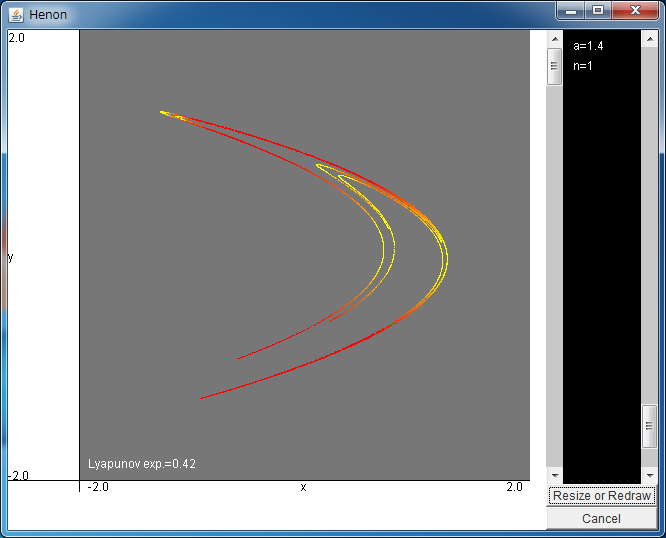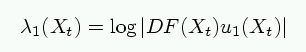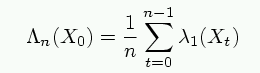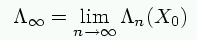# Attractor of Hénon MapYou can expand the area by dragging the field with your mouse.

If the above application does not start, please install OpenJDK from adoptium.net.

Let us treat the Hénon map written as

xt+1=1-axt2+byt
yt+1=xt.

As the initial configuration, the parameters are set as a=1.4 and b=0.3.

Generally, the determinant of the Jacobian matrix of the map denotes the expansion rate of the volume per an iteration in the phase space, and, in the Hénon map, it is calculated to be -b, and |-b|=0.3<1; therefore, the Hénon map is a dissipative system. Using the terms in physics, the energy of the system dissipates.

By taking the limit of small expansion rate, i.e., b=0, a one-dimensional map xt+1=1-axt2 is obtained. This map can be transformed into the logistic map. Although the logistic map has various properties of chaos in dissipative systems as shown in previous pages, it has a weak point that it is a one-dimensional map and therefore its attractor does not have fractal properties. This is because the limit of small expansion rate (b=0) is taken. Although the bifurcation diagram of the logistic map has fractal properties, the bifurcation diagram is a different concept from the attractor. The simplest model which shows an attractor with fractal properties, namely, strange attractor, is the Hénon map treated in this page.

To confirm the fractal properties of the attractor, let us expand the field with your mouse.
You will see that a seemingly single string is composed of multiple strings.
Theoretically, such a structure will be seen in the infinitesimal areas, but few points will be observed when you expand the area for several times,
because the number of time-iteration is finite.

Moreover, you can change the value of the parameter a with the right bar.
As the value of a is changed, the bifurcation phenomenon takes place like the logistic map,
and you can observe the periodic solutions, n-band chaos, and so on.

To judge whether the dynamics is chaos or not, the largest Lyapunov exponent is often used,
and this simulator also calculates this value and shows it in the field. If the dynamics is chaotic, the largest Lyapunov number take positive values.

The definition of the Lyapunov exponent is given in the following.
First, let us define the orbital expansion rate of the Hénon map Xt+1 = F (Xt) at the point Xt+1= (xt, yt) aswhere DF(Xt) is the Jacobian matrix of the map at Xt and u1(Xt) is a unit vector tangent to the attractor at Xt.

Using it, the coarse-grained expansion rate is defined aswhere the orbital expansion rate is averaged over n points.
The attractor of the above simulator is colored based of the value of the coarse-grained expansion rate, and it will be explained later.

By taking the infinitely large n limit, we can obtain the largest Lyapunov exponent asThe largest Lyapunov exponent takes constant value for almost all the initial points X0.
This simulator calculates the Lyapunov exponent by taking the average over more than 10000 iterations.
When the size of attractor is changed, or when "Resize or Redraw" button is pressed,
the Lyapunov exponent is re-calculated, and this value slightly changes for each trial.
It is because the average number is finite.

Though the Lyapunov exponent does not depend on the initial point X0, the coarse-grained expansion rate does.
The attractor of the above simulator is colored based on this value.
The yellow means that the coarse-grained expansion rate is small, and the red means large values.

In the initial condition, the value of n is 1, thus it is identical with the orbital expansion rate.
At the curves of the attractor, the orbital expansion rate takes negative values, thus they are colored with yellow.
And the sharp area of the attractor also form a curve, as you can see when you expand it, thus it is also yellow.

When you enlarge the value of n, the coarse-grained expansion rate takes in the information of the attractor, and takes various values on the attractor.
For example, please change the value of n to 5, and press the "Resize or Redraw" button. A few yellow areas will be observed.
These points pass the curve of the attractor in the following 5 iterations.

More specially speaking, the yellow area reflects the trajectory of the point of tangency of stable manifold and the attractor.
The stable manifold is introduced in "Attractor of Hénon map" gallery.
Thus, the statistical properties of the coarse-grained expansion rate reflects the phase space structure of the attractor.

By the way, for large n, the coarse-grained expansion rate is close to the Lyapunov exponent, thus the color of attractor will be uniform.